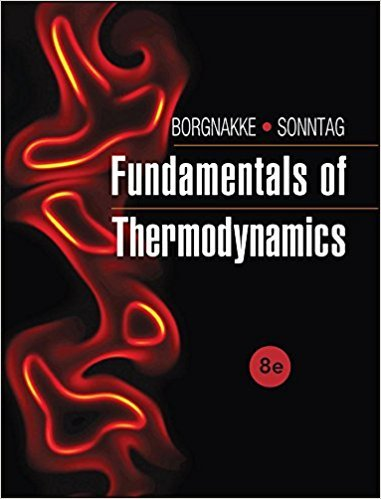# We want to find the change in u for carbon dioxide between## Problem 130HP Chapter 3

Fundamentals of Thermodynamcs | 8th Edition

• 2901 Step-by-step solutions solved by professors and subject experts
• Get 24/7 help from StudySoup virtual teaching assistantsFundamentals of Thermodynamcs | 8th Edition

4 5 0 274 Reviews
30
3
Problem 130HP

We want to find the change in u for carbon dioxide between 600 K and 1200 K.

a. Find it from a constant Cv0 from Table A.5.

b. Find it from a Cv0 evaluated from the equation in Table A.6. at the average T.

c. Find it from the values of u listed in Table A.8.

TABLE A.5

Properties of Various Ideal Gases at 25°C, 100 kPa*(SI Units)

 Gas ChemicalFormula Molecular Mass(kg/kmol) R(kJ/kg-K) ρ (kg/m3) Cp0(kJ/kg-K) Cv0(kJ/kg-K)Steam H2O 18.015 0.4615 0.0231 1.872 1.410 1.327 Acetylene C2H2 26.038 0.3193 1.05 1.699 1.380 1.231 Air — 28.97 0.287 1.169 1.004 0.717 1.400 Ammonia NH3 17.031 0.4882 0.694 2.130 1.642 1.297 Argon Ar 39.948 0.2081 1.613 0.520 0.312 1.667 Butane C4H10 58.124 0.1430 2.407 1.716 1.573 1.091 Carton dioxide CO2 44.01 0.1889 1.775 0.842 0.653 1.289 Carton monoxide CO 28.01 0.2968 1.13 1.041 0.744 1.399 Ethane C2H6 30.07 0.2765 1.222 1.766 1.490 1.186 Ethanol
Step-by-Step Solution:

Solution 130HP

Part (a)

Step 1 of 6:

We are going to find the change in the internal energy of Carbon dioxide gas for constant specific heat found in the table A.5.

The temperature T1 = 600 K

The temperature T2 = 1200 K

The value of specific heat at constant volume from the table (Cv0) = 0.653 kJ/kg.K

The change in the internal energy is given byInserting the valuesThe change in the internal energy is 391.8 kJ/kg.K.

Part (b)

Step 2 of 6:

We are going to find the specific heat from the equation given in the table A.6. The temperature is the average temperature from the part (a).

The temperature TaveThe average temperature is 900 K.

The equation for Cv0 is given byStep 2 of 6:

The value θ is expressed asThe values of C0, C1, C2, and C3 are taken from the table A.6

C0 = 0.45

C1 = 1.67

C2 = -1.27

C3 = 0.39

From the table A.5,  The gas constant for CO2 (R) = 0.1889 kJ/kg.K

Step 3 of 6:

Putting the numerical values in the equation (2)The specific heat for constant volume is given byStep 4 of 5

Step 5 of 5

##### ISBN: 9781118131992

Unlock Textbook Solution

We want to find the change in u for carbon dioxide between

×
Get Full Access to Fundamentals Of Thermodynamcs - 8th Edition - Chapter 3 - Problem 130hp

Get Full Access to Fundamentals Of Thermodynamcs - 8th Edition - Chapter 3 - Problem 130hp

I don't want to reset my password

Need help? Contact support

Need an Account? Is not associated with an account
We're here to help